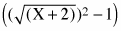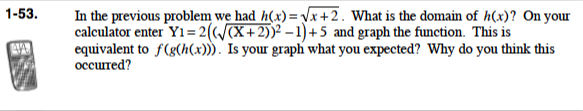### Home > PC > Chapter 1 > Lesson 1.1.4 > Problem1-53

1-53.In the previous problem we had h(x) =. What is the domain of h(x)? On your calculator enter Y1 = 2) + 5 and graph the function. This is equivalent to f(g(h(x))). Is your graph what you expected? Why do you think this occurred? Homework Help ✎The graph does not give a full line. The line starts at (−2,3) and then follows the line y = 2x + 7.
Since h(x) is defined for only values of x ≥ −2, the composite function is only defined for x ≥ −2.